#Function Repository Resource:

# SquareSpiralPoints

Get the coordinates of the points on a square spiral with sides parallel to the axes

Contributed by: George Beck
 ResourceFunction["SquareSpiralPoints"][n] gives the list of coordinate pairs on a square spiral with n sides starting at the origin.

## Details and Options

The list starts at the origin and goes up, left, down, right and repeats.

## Examples

### Basic Examples

Here are the points on the first four sides of the spiral:

 In:=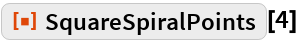Out=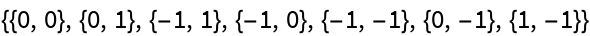This shows the sequence of points in order on the first six sides:

 In:=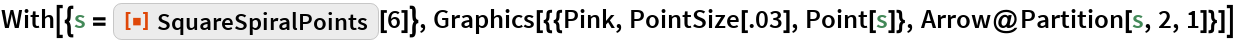Out=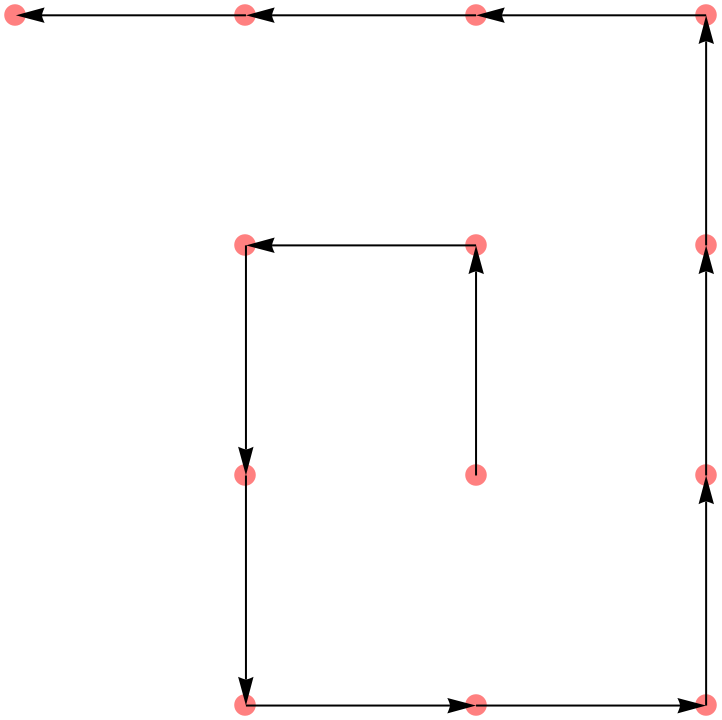Forty black sides with 20 red sides overlaid:

 In:=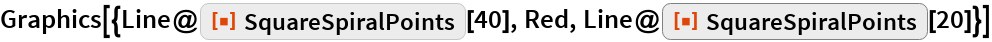Out=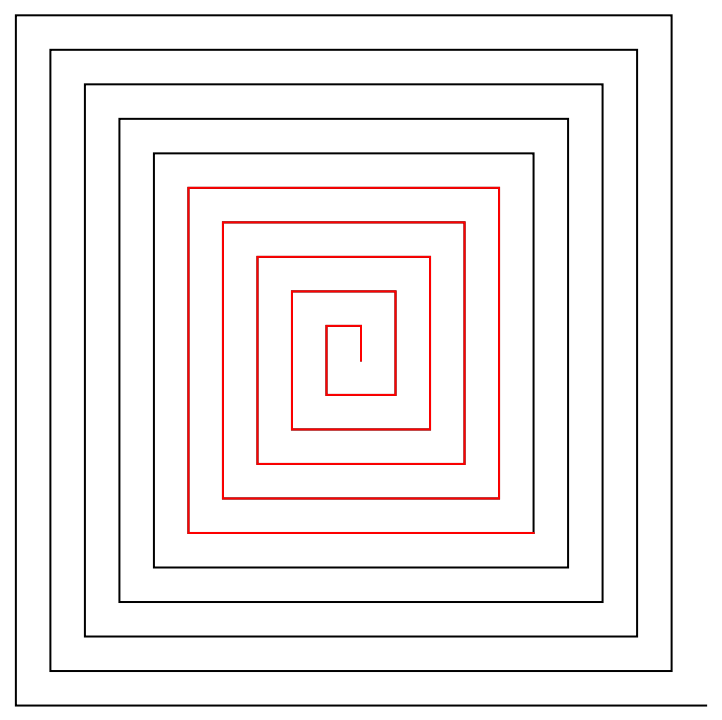The number of points in n sides:

 In:=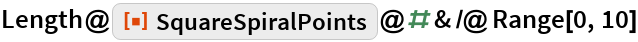Out=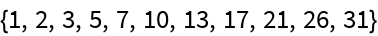In:=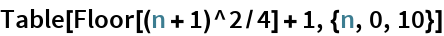Out=### Neat Examples

This finds the coordinate pairs that are a prime distance along the square spiral:

 In:=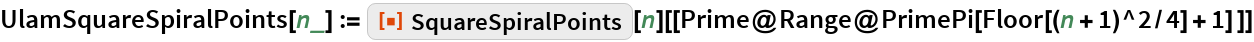The larger points correspond to the primes 2, 3, 5, 7, 11, 13:

 In:=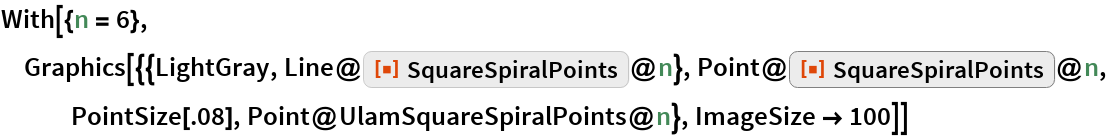Out=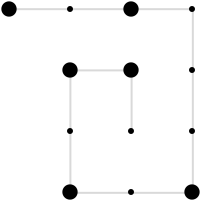About 12% of the numbers up to 10101 are prime:

 In:=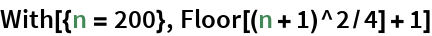Out=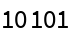In:=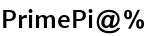Out=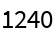Here are the first 1240 primes plotted along the square spiral:

 In:=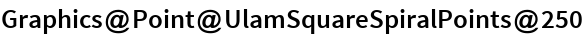Out=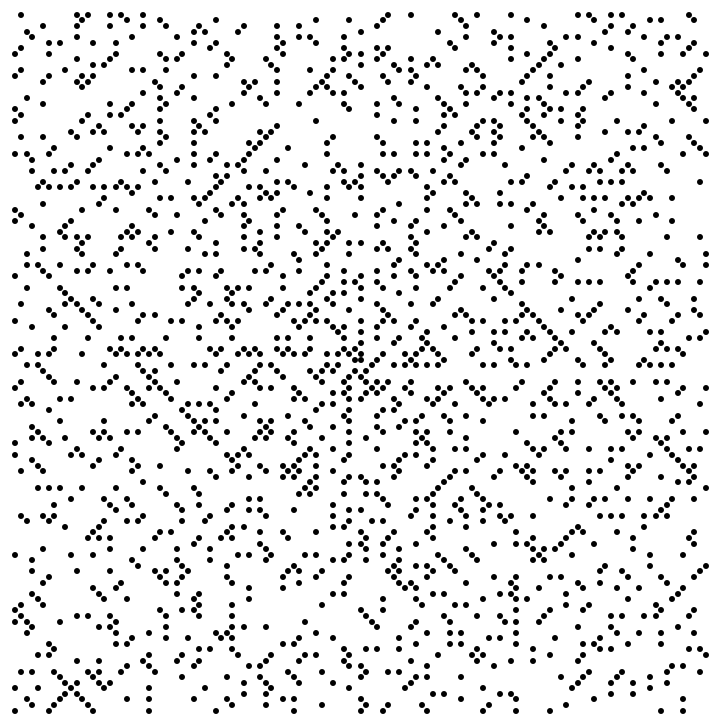About 11% of the numbers to 10101 are lucky:

 In:=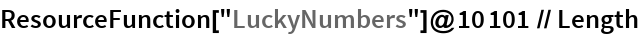Out=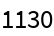This finds the coordinate pairs that are at lucky number distances along the square spiral:

 In:=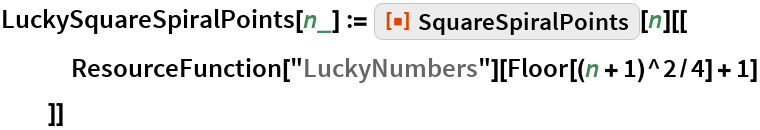Here are the first 1130 lucky numbers plotted along the square spiral:

 In:=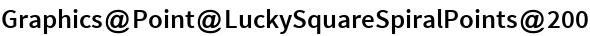Out=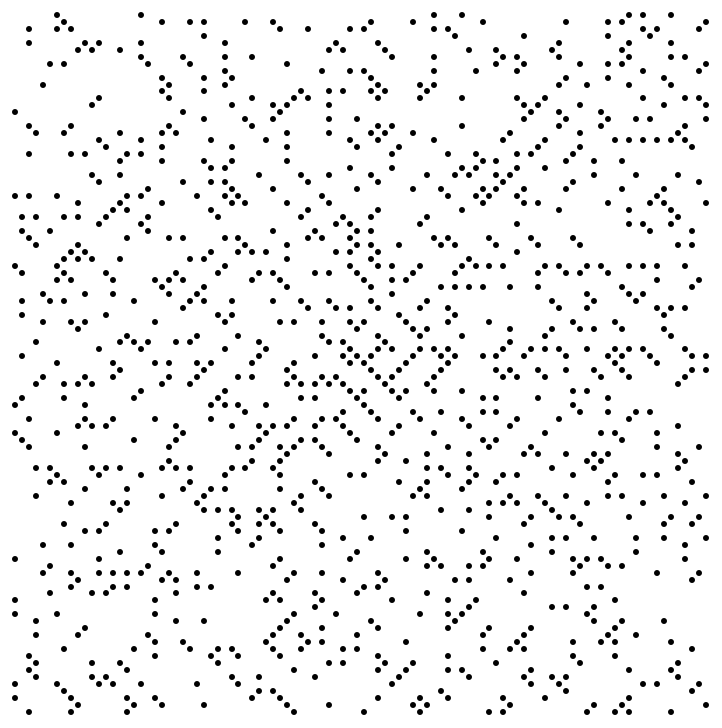## Requirements

Wolfram Language 11.3 (March 2018) or above